## MISC

### Questionnaire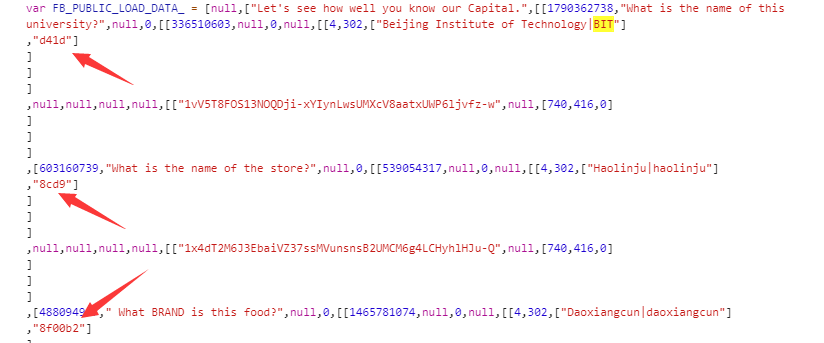### babyweb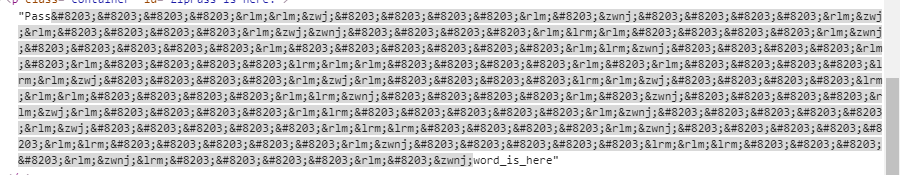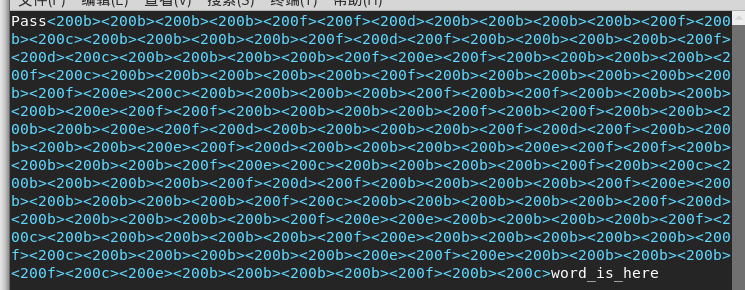``````f=open('f14g.png','r').read()
fi=open('out.png','w')
fi.write(f[::-1])
fi.close()``````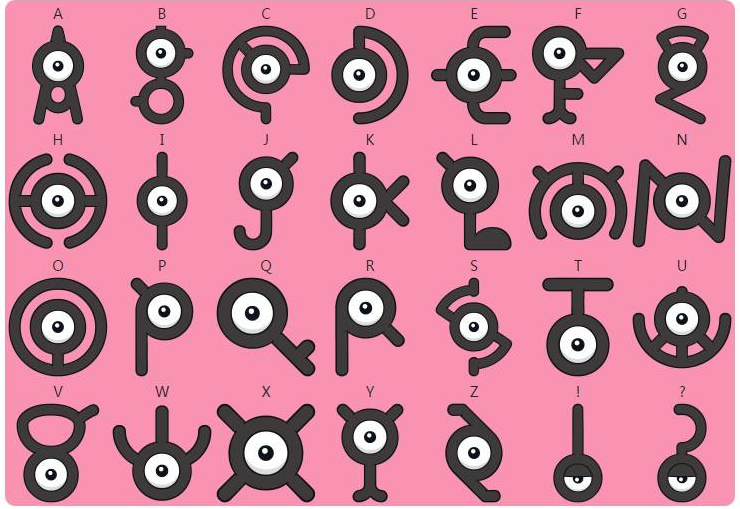### testyournc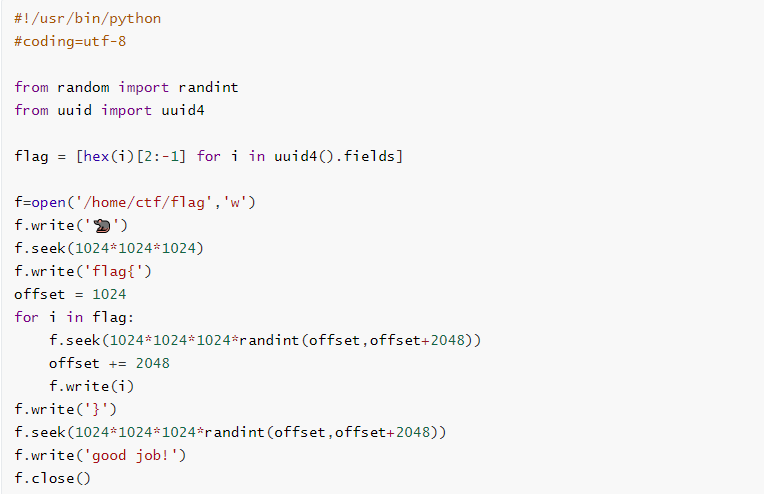``````a=open('flag')
a.seek(1024*1024*1024)
for i in range(1024,13428):
a.seek(1024*1024*1024*i)
continue
else:
a.seek(1024*1024*1024*i)
print(i)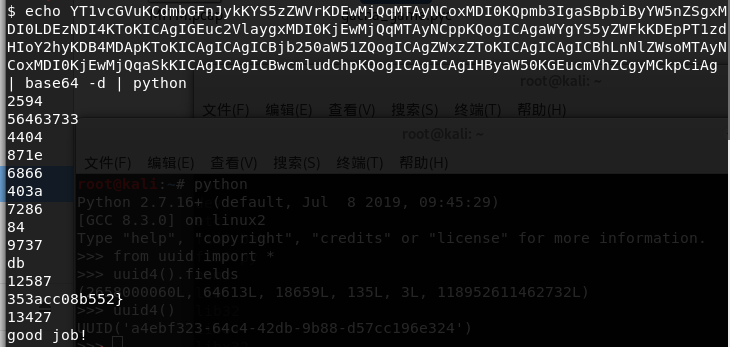### manual

ssh连上靶机，可以看到欢迎界面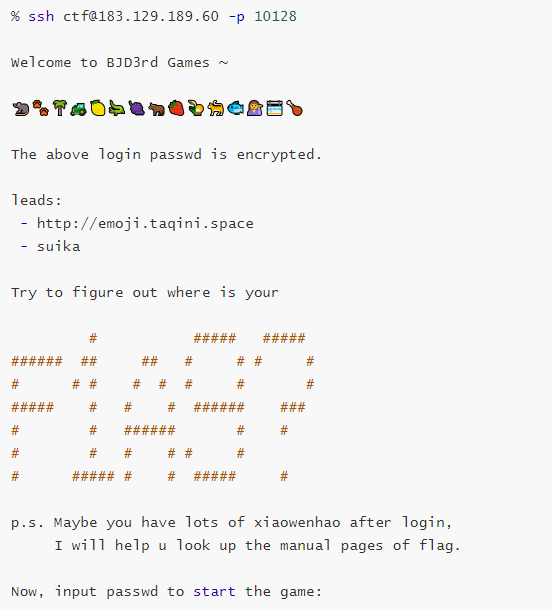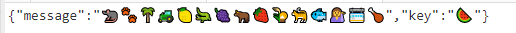``https://codemoji.org/share.html?data=eyJtZXNzYWdlIjoi8J%2BQgPCfkL7wn4y08J%2BanPCfjYvwn5CK8J%2BNh%2FCfkILwn42T8J%2BOkfCfkIjwn5Cf8J%2BSgfCfmp%2Fwn42XIiwia2V5Ijoi8J%2BNiSJ9``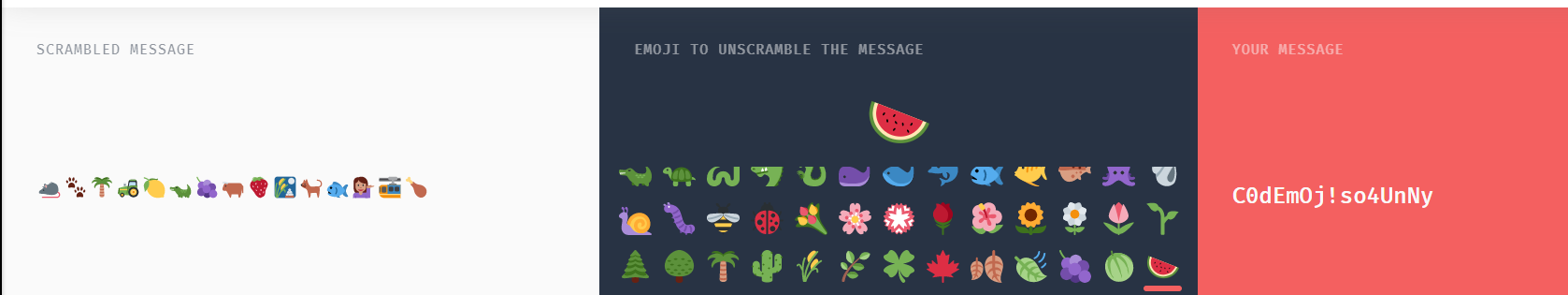（未完待续ing……）

## CRYPTO

### bbcrypto

``````# -*- coding:utf-8 -*-
import A,SALT
from itertools import *

def encrypt(m, a, si):
c=""
for i in range(len(m)):
c+=hex(((ord(m[i])) * a + ord(next(si))) % 128)[2:].zfill(2)
return c

if __name__ == "__main__":
m = 'flag{********************************}'
a = A
salt = SALT
assert(len(salt)==3)
assert(salt.isalpha())
si = cycle(salt.lower())
print("明文内容为：")
print(m)
print("加密后的密文为：")
c=encrypt(m, a, si)
print(c)
#加密后的密文为：
#177401504b0125272c122743171e2c250a602e3a7c206e014a012703273a3c0160173a73753d``````

``````a = 57
for i in range(97,123):
if hex((ord('f')*a + i) % 128)[2:].zfill(2) == '17':
print ('1:'+chr(i))
if hex((ord('l')*a + i) % 128)[2:].zfill(2) == '74':
print ('2:'+chr(i))
if hex((ord('a')*a + i) % 128)[2:].zfill(2) == '01':
print ('3:'+chr(i))``````

``````from binascii import unhexlify
from gmpy2 import *
from itertools import *

def decrypt(c, a, si):
m = b''
for i in range(len(c)):
m += bytes.fromhex(hex((c[i] - ord(next(si)) + 128) * invert(a,128) % 128)[2:].zfill(2))
return m

c = '177401504b0125272c122743171e2c250a602e3a7c206e014a012703273a3c0160173a73753d'
c = unhexlify(c)
a = 57
salt = 'ahh'
si = cycle(salt.lower())
print(decrypt(c, a, si))``````